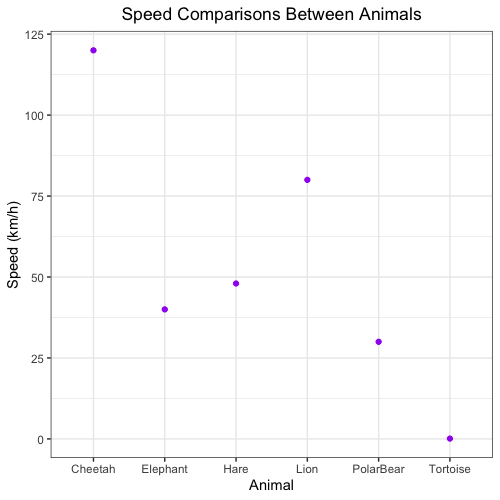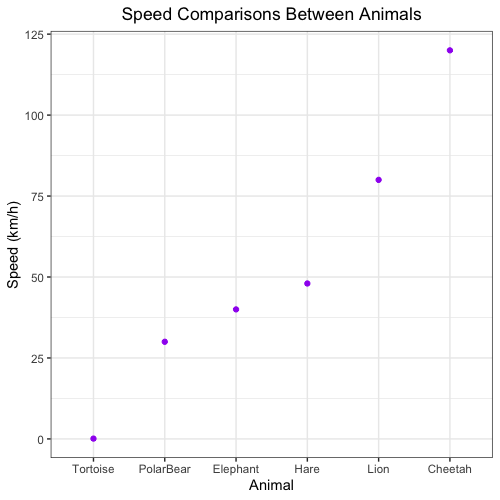# Plotting Practical

1. Add an additional column, called `animal_names`, in the `animals` data frame. This column stores the row names of the `animals` data frame. (NOTE: the original row names could stay as it is.)2. Use ggplot2 to plot the animal names (x-axis) versus the speed of the animal (y-axis) in `animals` using a scatterplot. Customize the plot to display as shown below.3. We decide that our plot would look better with the animal names ordered from slowest to fastest. Using the `animals` data frame, reorder the animals on the x-axis to start with the slowest animal on the left-hand side of the plot to the fastest animal on the right-hand side of the plot by completing the following steps:

a. We want to turn the `animal_names` column of `animals` into a factor and specify the levels as from slowest to fastest. Note: this step is crucial, because ggplot2 uses `factor` as plotting order, instead of the order we observe in data frame. To do this, use the `reorder()` function to order the rows by speed from slowest to fastest.

Note1: In the `reorder()` function, the first argument is the categorical variable to be reordered (in our case, `animal_names`), and the second argument is the variable in which the ordering is based on (in our case, `speed`). The `reorder()` function is used together with `with` function here (check the example of `reorder()` function).

Note2: An alternative solution is to manually specify the levels, based on the speed. However, when the dataset is big, this method is not feasible.

b. Re-plot the scatterplot with the animal names in order from slowest to fastest.Note: If you are interested in exploring other ways to reorder a variable in ggplot2, refer to this post.

4. Save the plot as a PDF called `animals_by_speed_scatterplot.pdf ` to the `results` folder.